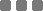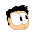Saturday, June 6, 2015

Math: Pattern of Squared Number Which Ends with 5

Kinda awkward title.
It's the pattern in decimal.
Shall we?
Let's take a look at this multiplication table
5
x
5
=
25
15
x
15
=
225
25
x
25
=
625
35
x
35
=
1225
45
x
45
=
2025
55
x
55
=
3025
65
x
65
=
4225
75
x
75
=
5625
85
x
85
=
7225
95
x
95
=
9025
105
x
105
=
11025
115
x
115
=
13225
...
1005
x
1005
=
1010025
...
5005
x
5005
=
25050025
...
et cetera

The 25
As you can see above, any number which ends with 5 if squared will have 25 as the "tail".

The yellow colored numero
I put yellow color for the "opening" number sequence because it's interesting.

Let's see the `15 x 15` line.
If we isolate the first number, which is 1, and we do this
1 x (1 + 1) = 2

It yields 2, the opening of 15² result, 225.

Another example, the `45 x 45` or `45²`.
We take the first number, which is 4, and we do the same technique
4 x (4 + 1) = 20

20 is the starting sequence (before the ending, 25) of the result, 2025.

Last example, the `5005 x 5005` or `5005²`.
500 x (500 + 1) = 250500
, which is also the first sequence of the result, 25050025.

In conclusion
For speed "squaring" a number which ends with 5, we can do this:
• The first number sequence:
the_first_number_before_5 x (the_first_number_before_5 + 1)
• The last (or the tail) will always be 25. We can just attach it to the first number sequence.

Usage
Let's find the result of 75².
• The first number sequence
• The tail is 25.
Then the result of 75² is 5625.

Number with decimal point?
Let's use the 75 sequence, but it's 0.75 now.

Calculate the result of 0.75² !
• Take the 7. So the first number sequence
• The tail is always 25.
• Last step is to count how many digits after the decimal point.
We have 0.75 (two digits) squared. Then we'll have 4 digits behind the thingy.
Then the result of 0.75² is 0.5625.

It sort of happens too in other number systems. But then, my brain hurts. So, thanks for visiting.Math: Pattern of Squared Number Which Ends with 5
https://monkeyraptor.johanpaul.net/2015/06/math-pattern-of-squared-number-which_6.html

1.Hi Johan,

That's some very keen thinking and a neat trick you have there! There's also another pattern: each number that we're squaring is an odd multiple of 5 (that is, 5*1, 5*3, 5*5,...), which we can write as (5*(2n-1))^2. Therefore, the prefactor must depend on which n we choose. If we pair up the first few n's with the prefactors, we find 1:0, 2:2, 3:6, 4:12, 5:20, etc. The relationship is that each n is multiplied by (n-1) (ex: 1*(1-1) = 0, 2*(2-1) = 2, 3*(3-1) = 6). Of course, the prefactors are multiples of 100, so we multiply by 100. Altogether we find that (5*(2n-1))^2 = 100*n*(n-1) + 25, which we could simplify to (2n-1)^2 = 4*n*(n-1) + 1.

Best,

Another Math Wizard

1.Wow that's really structured. I dig it!

Tell me what you think...